## 2.12.4 Multiple Independent Poisson Processes

Suppose that there are two Poisson processes operating independently, with arrival rates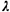1 and2 respectively. N1(t) and N2(t) are the respective cumulative numbers of arrivals through time t. Then the combined or pooled process has a cumulative number of arrivals equal to N(t) = N1(t) + N2(t). A fundamental property of independent Poisson processes is that their pooled process is also a Poisson process with arrival-rate parameter equal to the sum of the individual arrival rates. Thus, N(t) has a Poisson distribution with mean (1 +2)t. This result extends in the obvious way to more than two independent Poisson processes. There are many ways to prove this result, but the simplest is just to observe that the pooled process satisfies each of the four postulates of the Poisson process.

We are often confronted with the following question: Given two independently operating Poisson processes with rate parameters1 and2 respectively, what is the probability that an arrival from process 1 (a "type 1" arrival) occurs before an arrival from process 2 (a "type 2" arrival)? To solve this problem, let the two arrival times of interest be denoted by X1 and X2, for processes 1 and 2, respectively. We want to compute P{X1 < X2}. Invoking our knowledge of Poisson processes, we know that the pdf's for X1 and X2 are negative exponentials with means1-1 and2-1, respectively. Thus, because of independence, the joint pdf is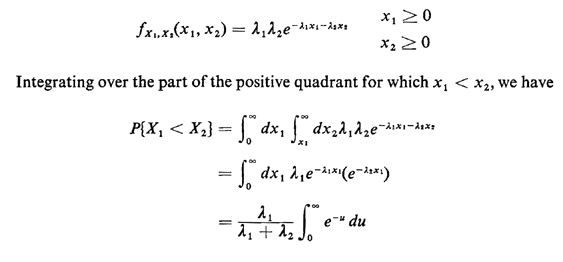or, since the integral equals 1,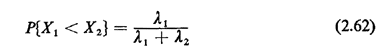This result makes sense intuitively: The probability that a type 1 arrival occurs before a type 2 arrival is equal to the fraction of the pooled arrival rate comprising type 1 arrivals.

This important result can be derived in a number of other ways, as well. Suppose that we are awaiting the first Poisson-type arrival and finally we are given the conditional information that the arrival occurred in the interval [t, t + dt]. Let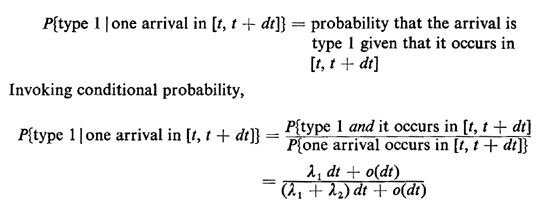Since dt is infinitesimal, we can ignore the o(dt) term, thereby obtaining1/(1 +2) for the conditional probability, conditioned on the infinitesimal arrival interval. We uncondition the probability by integrating over all possible arrival intervals, weighted by the probability of their occurrence,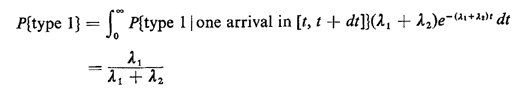Yet a third way of deriving the result involves consideration of a long time period of length T. During that period the expected total number of arrivals is (1 +2))T; the expected number of type 1 arrivals is1T. Thus, roughly speaking, the fraction of total arrivals that is type 1 is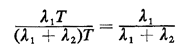a result in agreement with the more formally derived (2.62).

A final point about this result: in examining a Poisson process we occasionally only have to look at the arrival instants, distinguishing between, say, type 1 and type 2 arrivals, not caring about the exact times of their occurrence. Then, because of the no-memory property of Poisson processes, each arrival instant becomes an independent Bernoulli experiment, with probability of a type 1 arrival equal to2/(1 +2) and of a type 2 equal to1/(1 +2). Thus, when considering questions of the types of Poisson arrivals occurring (and not their times), we may invoke ideas of Bernoulli experiments which often lead to geometric distributions, binomial distributions, and other pmf 's associated with independent discrete random variables.

We apply most of our results for a Poisson process to a "pedestrian crossing" problem at the end of the chapter.